Mathematics
Easy

Question

# If a function f(x) represents the height of the football when kicked and f(x) =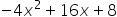What is the quadratic equation if the height of the ball after the kick is 20 m?

##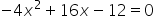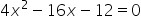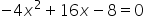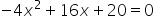Hint:

## The correct answer is:### Step 1 of 1:We have given a function f(x) =f(x) =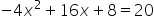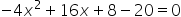The discriminant is the part of the quadratic formula found within the square root.

### Related Questions to study#### With Turito Foundation.#### Get an Expert Advice From Turito.